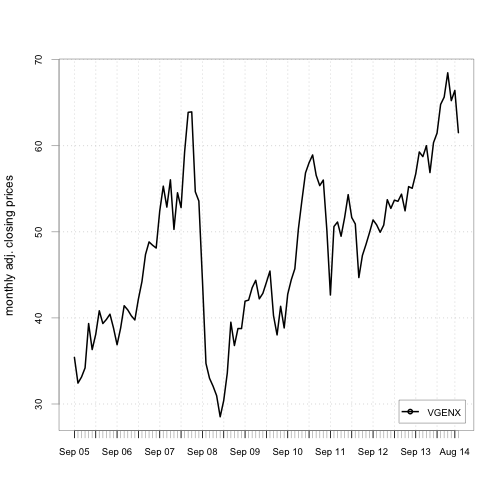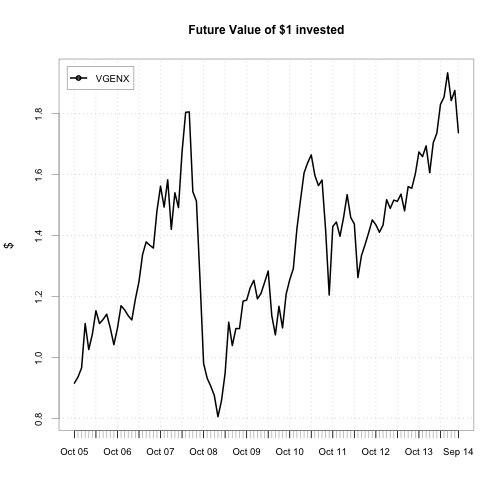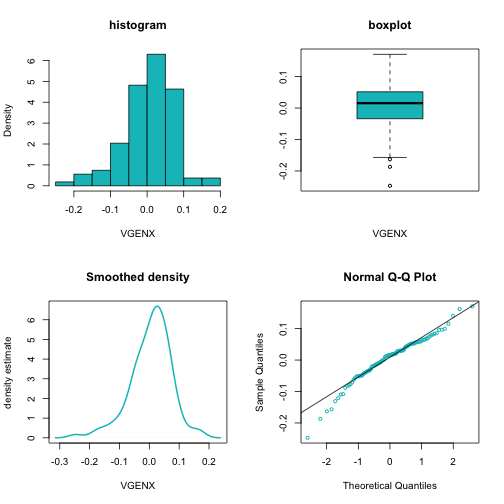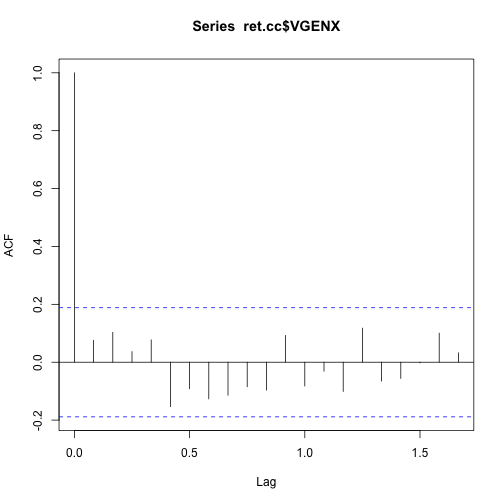Analyze Stock Price Data Using R, Part1

Master R

Crude oil has been hammered. Yesterday, it hit the lowest price in 2 years. Being a contrarian, I smell the opportunity to buy. My interest is a vanguard energy fund (VGENX), which has 95% of its assets invested in oil or oil related sectors. Let’s first take a look at its prices and returns histories since Sept 2005.

Step 1. Load libraries and helper functions

Step 3. Change the class of the time index to yearmon.

Step 4. Calculate continuously compounded returns.

Step 5. Plot prices.Step 6. Plot cumulative returns.Step 7. Plot the distribution of cc returns.The histogram, boxplot and the smoothed density curve show the cc returns are slightly left skewed. The qqplot shows their distribution has fatter tails.

Step 8. Compute all of the relevant descriptive statistics

Indeed, we see the cc returns have a negative skewness and an excess kurtosis of 1.55 compared to the normal distribution.

Step 9. Run the Jarque Bera test to see if the cc returns are normal

Because the p-value is extremely small, we have strong evidence to reject the null hypothesis that the continously compounded monthly returns for VGENX are normally distributed.

Step 10. Plot autocorrelations over time lagsThe monthly cc returns doesn’t appear to be correlated over time.

Step 11. Compute the annualized continuously compounded mean return and volatility

Step 12. Compute the annualized simple mean return